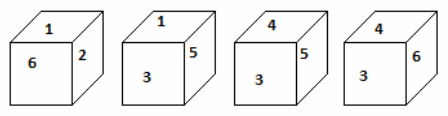# Cubes & Dices

Cube
Most of the aptitude exam have consist of this kind of dice problems. Here we will discuss methods to solve such problems but before that let have a look on CUBE
·       It has 6 sides/faces , 8 corners and 12 edges.
·       Length of all the side are equal
·       If x be the length of side then,
o    Area of all surfaces = 6x2
o    Volume = x3
·       When a bigger cube is cut in N equal small size cube then Area of Bigger Cube = N× Area of Smaller Cube
Let’s understand with an example
A cube is painted blue on all the faces. Then cut into 27 cubes of equal size. Length of side of bigger cube is 6 m.

Area of Bigger Cube = N× Area of Smaller Cube
= 125× Area of Smaller Cube
53 = 125×X3
Size of smaller cube  X = 1

Since the side of smaller cube is 1/ 5th side of larger cube , hence there would be five layers of smaller cubes.Observations:
In each layer :
9 middle cubes painted only one face
4 cubes at the corner painted three face
Remaining 12 cubes painted 2 faces.
In three middle layers:
9 middle cubes painted no color
4 cubes at the corner painted two color
Remaining 12 cubes are painted one face
Formulas :
For Number of layer = n
·         No of cubes painted no color = (n-2)3
·       No of cubes painted only one face = (n-2)2×6
·       No of cubes painted only two faces = (n-2)× 12
·       No of cubes painted three faces = 8(corner cubes only)
·       No of cubes painted only one face = (n-2)2×6

Dice

In these types of problem some different positions of a DICE would be given, based on that we are ask to find the numbering on different faces.Example:
From the positions of a cube are shown below, Which letter will be on the face opposite to face with '1'?Explanation:
5 and 6 are on the opposite face.
Only number remains is 4.
Hence the number opposite to the face of 1 would be 4Thanks for reading Cubes & Dices

←Previous
« Prev Post
Next→
Next Post »

Video Lectures